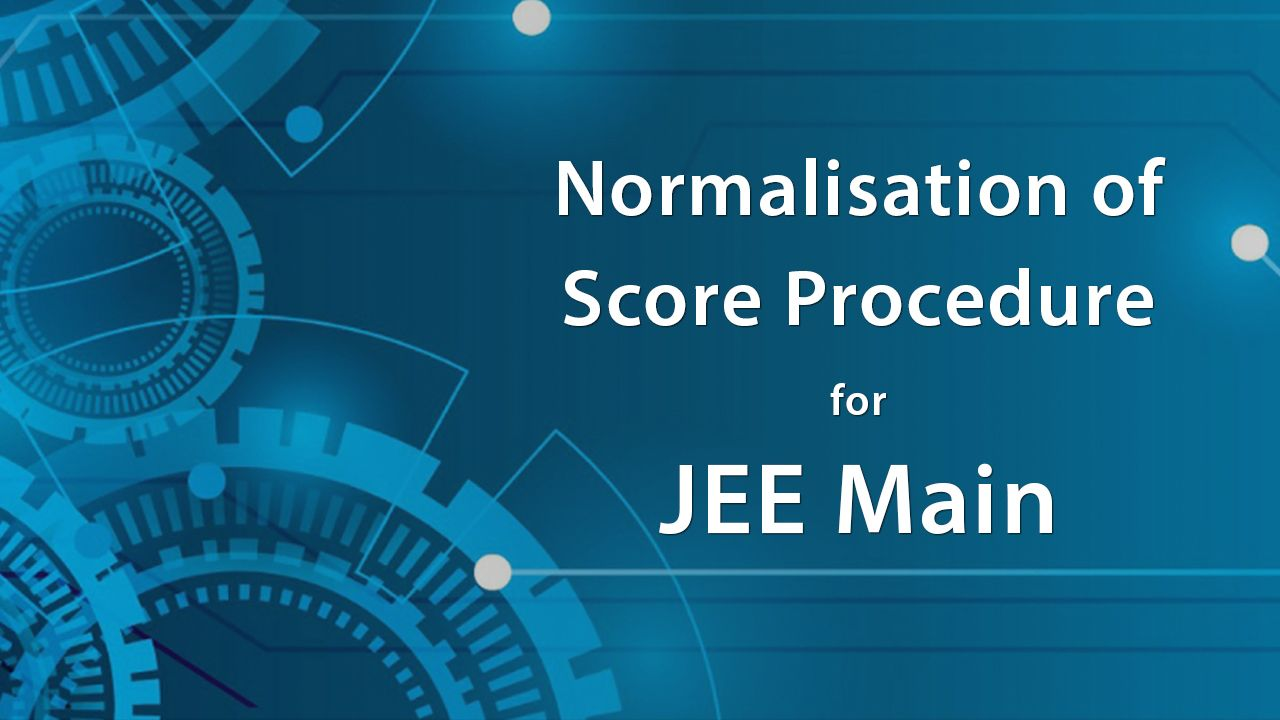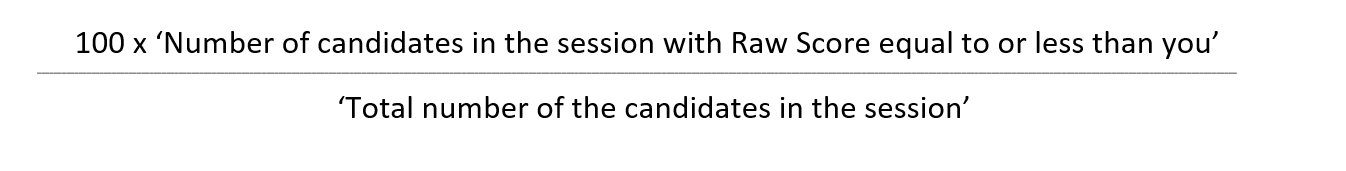Success is thrilling.

Ensure it with CareerOrbits.

# JEE Main Normalisation procedure for calculation of percentile score for JEE Main 2021 ExamIn 2019, NTA has re-introduced the JEE Main normalisation procedure to ensure each and every student gets a fair chance for scoring good marks. From 2019, JEE Main exam will be conducted four times in the months of February, March, April and May on multiple dates and in two sessions per day. Now as the JEE Main examination becomes a multi-session examination, there is a need to calibrate the marks scored by students in the examinations so that the best and most deserving JEE Main aspirants make it to the prestigious institutes like IIITs, NITs as well as be eligible to sit for JEE Advanced.

Although the NTA has made all the efforts to maintain the level of questions across the different question papers, the difficulty level of some questions might not be exactly the same. As a result, some students might attempt relatively difficult question paper compared to other JEE Main aspirants and these aspirants might not be able to score well in those difficult questions leading to a lower score compared to students who get a relatively easier paper.

So, NTA has adopted the ‘Normalisation Procedure based on Percentile Score’ to overcome this situation and that no student appearing for the exam will be at any disadvantage.

## Normalisation for JEE Main - What is Percentile Score?

Most of the JEE Main aspirants are not aware of how the Percentile is calculated and how it's different from ‘Percentage’. So, before explaining the JEE Main Normalisation procedure, the JEE Main aspirants should be clearly able to understand how “Percentile” works.

What is Percentage?

Throughout your school years, you have been assessed on the percentage format where the marks we score out of 100 are considered as the percentage.

So, if you had scored 85 marks out of 100 marks, then your percentage is 85%. But the method of calculating the percentile is absolutely different. Hence, you should not confuse with both the terms.

### What is JEE Main percentile score and how it is different?

JEE Main Percentile score is a relative score and reflects your performance compared to all the other students who have appeared in JEE Main 2021 exam. Hence, your score will indicate how many students who appeared in JEE Main have scored equal to or below you in your JEE Main Examination.

The student with the maximum marks in that JEE Main exam session will be the topper of that session and will be awarded 100 percentile. This would imply that 100% of the students who appeared in the JEE Main 2021 exam have scored equal to or less marks as the topper.

As over 10 lakh students appear in JEE Main each year, NTA has decided to calculate the JEE Main Percentile score to up to 7 decimal places to reduce ties and avoid the bunching effect. This will ensure that each student will have a unique percentile and this will reduce the chance of multiple students scoring the same percentile in the JEE Main exam.

Your JEE Main Percentile score will be calculated in the below manner:## JEE Main Normalisation Procedure - How to calculate percentile for JEE Main 2021

NTA will utilise the raw scores of the candidates appearing in one session to calculate the percentile score of these candidates for that particular session. So, if a student (say Student A) appears in JEE Main on 26th February, Shift-2, then his percentile score will be calculated based on the score of the other students who gave the exam with Student A.

### A detailed example on how to calculate normalised score for JEE Main

JEE Main Percentile Score is calculated based on the raw scores (which is the actual score of each candidate) and the number of candidates who appeared in the exam in the particular session.

So, if Student A appears in the exam in a particular session (say Day 1, Shift 1), then the scores will be based on the raw scores of the candidates from that session. The details of the total number of candidates appeared, highest and lowest raw scores are given below.

 Session Day and Shift No. of Candidates Raw Score Absent Appeared Total Best Worst Session 1 Day-1 and Shift-1 6465 65489 71954 346 -49

Here, in Session 1, there were total of 71,954 students who were supposed to appear for the exam. Now out of the total candidates only 65,489 students actually gave the exam.

As explained earlier the raw score is the actual score achieved by the candidate after considering the negative marking for each wrongly attempted question.

Normalisation process for JEE Main - How your percentile will be calculated if you score the highest marks in your respective JEE Main 2021 session

To calculate the percentile score for the candidate who has scored the highest raw score, all the candidates who have scored the highest marks in their respective sessions will have 100 Percentile as per the NTA Normalisation process for JEE Main.

The below example explains how the highest raw scores of each session will be normalised for calculating the JEE Main percentile Score.

 JEE Main Session Total Candidates Appeared Highest Raw Score No. of Candidates who scored equal to or less than the Highest Raw score 100 * Candidates who scored equal to or less than the Highest Raw score / Total Candidates Appeared = Percentile Score Session 1 65489 346 65489 100*65489 / 65489 = 100.0000000 Session 2 95165 330 95165 100*95165 / 95165 = 100.0000000 Session 3 24686 332 24686 100*24686 / 24686 = 100.0000000 Session 4 81321 335 81321 100*81321 / 81321 = 100.0000000

Normalisation process for JEE Main - How your percentile will be calculated if you score the lowest marks in your respective JEE Main 2021 session

If you end up scoring the lowest marks in the JEE Main session in which you appear, then your percentile score depends upon the total number of candidates who appeared in the JEE Main exam.

Below is an example of how the marks of the lowest scoring student will be calculated as per the JEE Main normalisation criteria prescribed by NTA.

 Session Total Candidates Appeared Lowest Raw Score No. of Candidates who scored equal to or less than the Lowest Raw score 100 * Candidates who scored equal to or less than the Lowest Raw score / Total Candidates Appeared = Percentile Score Session 1 65489 -49 1 100*1/65489 = 0.0015269 Session 2 95165 -38 1 100*1/95165 = 0.0010508 Session 3 24686 -44 1 100*1/24686 = 0.0040508 Session 4 81321 -39 1 100*1/81321 = 0.0012296

So, based on the understanding of the percentile, let’s calculate JEE Main Percentile Scores by taking a few random raw scores for Session 1 (Day 1 - Shift 2) in which 65,489 candidates appeared for the test.

 Candidate Raw score No. of Candidates who scored equal to or below the candidate’s raw score 100 * Candidates who scored equal to or less than the Candidate / Total Candidates Appeared = Percentile Score A 330 55165 100*55165/65489 = 84.2355205 B 243 42453 100*42453/65489 = 64.8246270 C 155 28034 100*28034/65489 = 42.8071890 D 87 10355 100*10355/65489 = 15.8118157 E -10 1687 100*1687/65489 = 2.5760051

## Additional Information about the normalisation of JEE Main score, calculation of percentile score and preparation of Merit List or Ranks

NTA will distribute the aspirants appearing in JEE Main across the different exam sessions in such a way that the number of candidates in each session is approximately equal.

For each of the JEE Main sessions, NTA will calculate the following results for each of the candidates:

1. Raw Marks for each subject - Physics, Chemistry and Mathematics

2. Subject-wise JEE Main Percentile score for Physics, Chemistry and Mathematics based on the raw score in the respective subjects

3. Total Raw Score

4. Overall JEE Main Percentile based on Total Raw Score

Each of the Subject-wise Percentiles and Overall Percentile score will be compiled for all the sessions separately and will be called NTA Scores. These will be used for the compilation of results and preparation of the overall Merit List / Ranking.

After the first attempt (February Attempt), NTA scores corresponding to the raw marks obtained by a candidate in each session will be calculated as per the procedure explained above.

For the declaration of result, the NTA Scores calculated for all the sessions in February Attempt (First Attempt) will be compiled. The result for JEE Main February attempt (first attempt) will only have the individual percentile scores for the three subjects – Mathematics, Chemistry and Physics as well as the total percentile score achieved in the February attempt. However, the ranks will not be declared.

NTA will follow the same process to calculate the scores for the April attempt of the JEE Main examination. Each candidate that appears in JEE Main April attempt will get the four percentile scores, i.e. three subject level scores (one each for Mathematics, Chemistry and Physics) and one total percentile score.

Now, NTA will work on the compilation of the Merit List or allocating Ranks to the students who appeared in the JEE main exam. NTA will now consider the best of your JEE Main percentile scores (from February, March, April and May 2021 attempt) to prepare the merit list and allocate the suitable rank to each student.

There may be some candidates with the same percentile score. To ensure that these ties are resolved, the candidates will be ranked in the following order:

• Higher Percentile score in Mathematics

• Higher Percentile score in Physics

• Higher Percentile score in Chemistry

• Candidates older in age

The sequence will be followed until the tie is resolved.

Once all the ties are resolved, NTA will prepare and publish the Final Ranking/Merit List will be prepared.